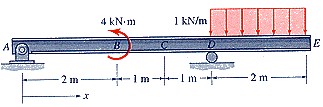### Create an Account

Home / Questions / Plot the shear diagram and the moment diagram for beam AEin Fig Plot these on paper Your i...

# Plot the shear diagram and the moment diagram for beam AEin Fig Plot these on paper Your instructor may ask tocollect themUse the Equilibrium Equation Method to obtain

Plot the shear diagram and the moment diagram for beam AEin Fig. P5.4-11. (Plot these on paper. Your instructor may ask tocollect them.)Figure P5.4-11.

Use the Equilibrium Equation Method to obtain and plotexpressions for V(x) andM(x), as illustrated in Examples 5.4 and5.5in the text. This problem may require several expressionsVi(x) for shear andMi(x) for moment. Therefore,you willneed to draw several free-body diagrams and solve the necessaryequilibrium equations to obtain these expressions. Draw therequested shear-force andbending-moment diagrams approximately toscale. Label all critical ordinates, including the maximum andmimimum values, and indicate the sections at which these occur.

Apr 27 2020 View more View LessSubscribe To Get Solution